Goseeko blog# What is Fourier Transform?

The Fourier transform simply states that any non-periodic signal which has finite area under the curve can be represented into integrals of the sines and cosines after being multiplied by a certain weight. The Fourier transform is given by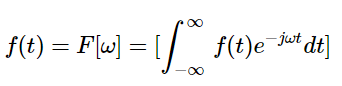The inverse is given as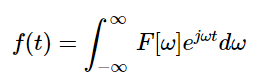## Conditions for Fourier Transform

We can find Fourier transform of a function if it satisfies Dirichlet’s condition. For the existence of its Fourier Transform. The conditions are

• The function must have finite maxima and minima.
• In a given interval of time the function should have finite discontinuities.
• The function must be integrable at a given interval of time.

## Discrete Time Fourier Transform

The sequence in discrete time can be represented by x[n] and the sequence terms are of complex exponential terms as shown below.

The DTFT sequence is as shown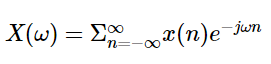The Inverse DTFT is as shownCondition for Convergence

The term x[n] in the below series is always summable irrespective of the fact whether the series converges or not.Then the sequence will have finite energy if it is absolutely summable and most importantly, finite energy sequence is not always absolutely summable.

## Properties

If x(t)↔ X(⍵) and the output y(t)↔Y(⍵). Then the properties of Fourier Transform are

Linearity property

Then this property follows the superposition theorem. Moreover the linearity property shows that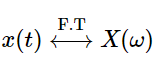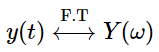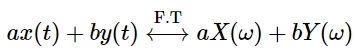Time Shifting Property

The time-shifting property means that a shift in time corresponds to a phase rotation in the frequency domain.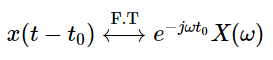Frequency Shifting Property

Secondly, This property shows that multiplication in frequency domain results in frequency shifting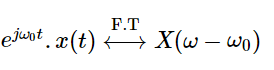Time Reversal Property

Lastly the time reversal property is as shown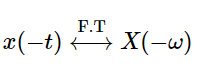Time Scaling Property

This property shows that if a function is expanded in time by a quantity a , the F.T is compressed in frequency by the same amount.

Hence, we can write the property is as shown in equation.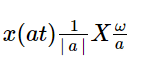Differentiation Property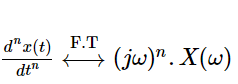Integration Property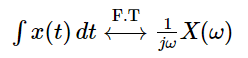Convolution Property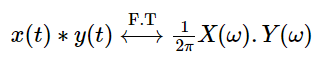## Application

Their main application is in  image compression, filtering and image analysis.

Interested in learning about similar topics? Here are a few hand-picked blogs for you!